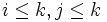# Directed union of subgroups is subgroup

## Contents

This article gives the statement, and possibly proof, of a basic fact in group theory.
View a complete list of basic facts in group theory
VIEW FACTS USING THIS: directly | directly or indirectly, upto two steps | directly or indirectly, upto three steps|

## Statement

### Verbal statement

The union of a nonempty directed set of subgroups of a group is again a subgroup.

### Statement with symbols

Suppose$G$ is a group,$I$ a nonempty directed set, and$H_i, i \in I$ is a collection of subgroups of$G$ indexed by$I$, such that$i \le j \implies H_i \le H_j$. Then, the subset of$G$ given by:$\bigcup_{i \in I} H_i$

is also a subgroup of$G$.

## Definitions used

### Directed set

A partially ordered set$I$ is termed directed if for any$i,j \in I$, there exists$k \in I$, such that$i \le k, j \le k$.

## Proof

Given:$G$ is a group,$I$ a nonempty directed set, and$H_i, i \in I$ is a collection of subgroups of$G$ indexed by$I$, such that$i < j \implies H_i \le H_j$.

To prove: The subset of$G$ given by:$\bigcup_{i \in I} H_i$

is also a subgroup of$G$.

Proof: We check the three conditions for a subgroup:

1. Identity element: Indeed, the identity element of$G$ is in all the$H_i$s, so it is in their union.
2. Inverse elements: Suppose$x$ is in the union. Then,$x \in H_i$ for some$i \in I$. Thus,$x^{-1} \in H_i$ (because$H_i$ is a subgroup). So,$x^{-1}$ is in the union.
3. Products: Suppose$x,y$ are in the union. Then,$x \in H_i$,$y \in H_j$ for some$i,j \in I$. By the directedness property, there exists$k \in I$, such that$i \le k, j \le k$. Thus,$H_i \le H_k$ and$H_j \le H_k$. In particular, both$x$ and$y$ are in the subgroup$H_k$. So, their product$xy$ is in$H_k$, so$xy$ is in the union.